## Breaking

CustomWritings.com - get an essay written for you by academic experts. Feel free to get writing assistance online.

## RD Sharma Solutions for Class 7 Maths Chapter 15 Properties of Triangles Free Online

Exercise 15.1 Page No: 15.4
1. Take three non-collinear points A. B and C on a page of your notebook. Join AB, BC and CA. What figure do you get? Name the triangle. Also, name
(i) The side opposite to ∠B
(ii) The angle opposite to side AB
(iii) The vertex opposite to side BC
(iv) The side opposite to vertex B.
Solution: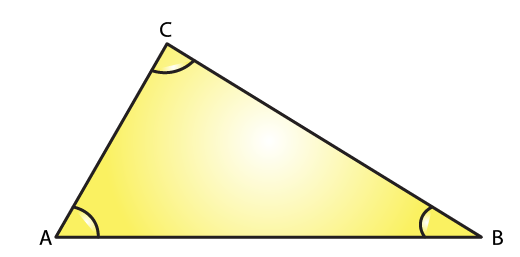(i) The side opposite to ∠B is AC
(ii) The angle opposite to side AB is ∠B
(iii) The vertex opposite to side BC is A
(iv) The side opposite to vertex B is AC
2. Take three collinear points A, B and C on a page of your note book. Join AB. BC and CA. Is the figure a triangle? If not, why?
Solution: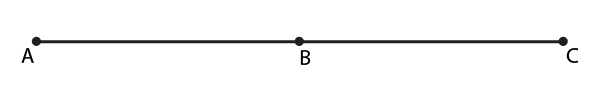No, the figure is not a triangle. By definition a triangle is a plane figure formed by three non-parallel line segments
3. Distinguish between a triangle and its triangular region.
Solution:
Triangle:
A triangle is a plane figure formed by three non-parallel line segments.
Triangular region:
Whereas, it’s triangular region includes the interior of the triangle along with the triangle itself.
4. D is a point on side BC of a △CAD is joined. Name all the triangles that you can observe in the figure. How many are they?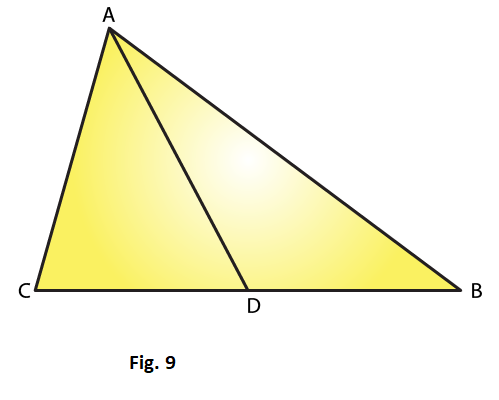Solution:
We can observe the following three triangles in the given figure
△ ABC
△ ACD
5. A, B. C and D are four points, and no three points are collinear. AC and BD intersect at O. There are eight triangles that you can observe. Name all the triangles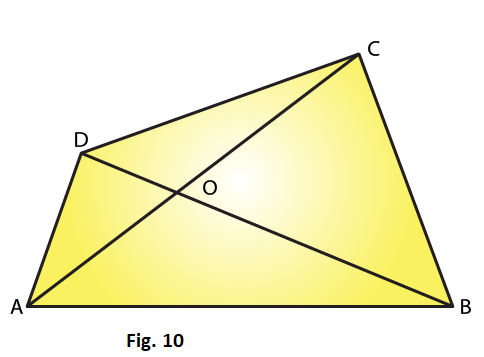Solution:
Given A, B. C and D are four points, and no three points are collinear
△ ABC
△ ABD
△ ABO
△ BCD
△ DCO
△ AOD
△ ACD
△ BCD
6. What is the difference between a triangle and triangular region?
Solution:
Triangle:
A triangle is a plane figure formed by three non-parallel line segments.
Triangular region:
Whereas, it’s triangular region includes the interior of the triangle along with the triangle itself.
7. Explain the following terms:
(i) Triangle
(a) Parts or elements of a triangle
(iii) Scalene triangle
(iv) Isosceles triangle
(v) Equilateral triangle
(vi) Acute triangle
(vii) Right triangle
(viii) Obtuse triangle
(ix) Interior of a triangle
(x) Exterior of a triangle
Solution:
(i) A triangle is a plane figure formed by three non-parallel line segments.
(ii) The three sides and the three angles of a triangle are together known as the parts or elements of that triangle.
(iii) A scalene triangle is a triangle in which no two sides are equal.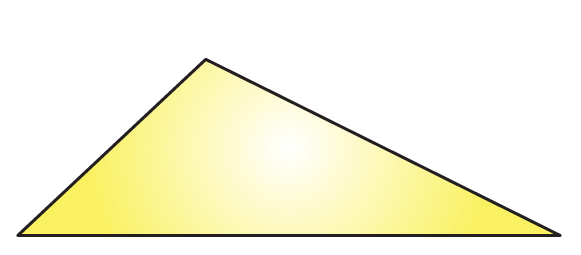(iv) An isosceles triangle is a triangle in which two sides are equal.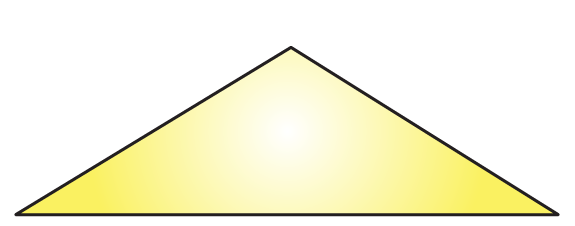(v) An equilateral triangle is a triangle in which all three sides are equal.(vi) An acute triangle is a triangle in which all the angles are less than 90o.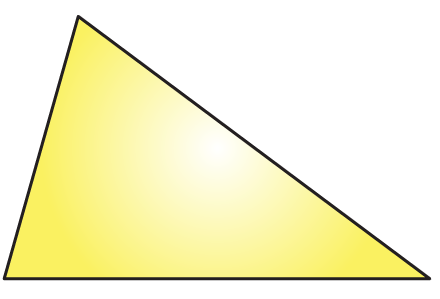(vii) A right angled triangle is a triangle in which one angle should be equal to 90o.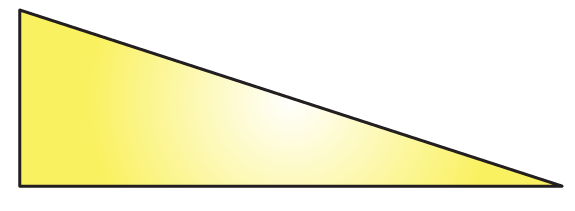(viii) An obtuse triangle is a triangle in which one angle is more than 90o.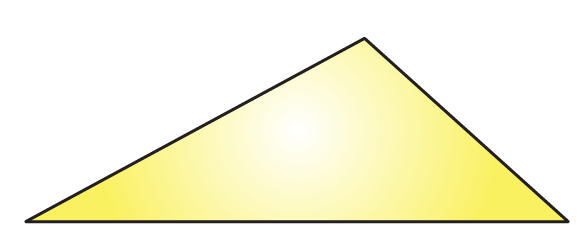(ix)  The interior of a triangle is made up of all such points that are enclosed within the triangle.
(x) The exterior of a triangle is made up of all such points that are not enclosed within the triangle.

8. In Fig. 11, the length (in cm) of each side has been indicated along the side. State for each triangle angle whether it is scalene, isosceles or equilateral: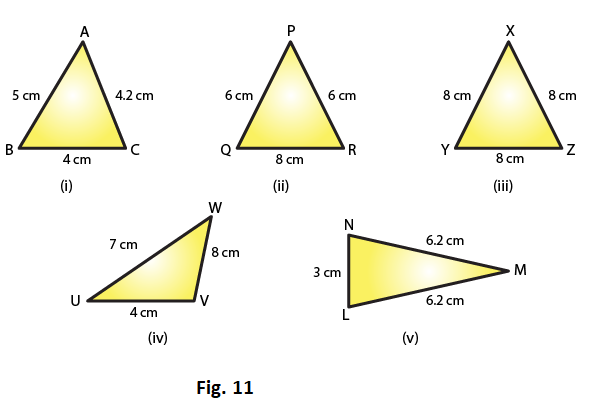Solution:
(i) The given triangle is a scalene triangle because no two sides are equal.
(ii) The given triangle is an isosceles triangle because two of its sides, viz. PQ and PR, are equal.
(iii) The given triangle is an equilateral triangle because all its three sides are equal.
(iv) The given triangle is a scalene triangle because no two sides are equal.
(v) The given triangle is an isosceles triangle because two of its sides are equal.
9. In Fig. 12, there are five triangles. The measures of some of their angles have been indicated. State for each triangle whether it is acute, right or obtuse.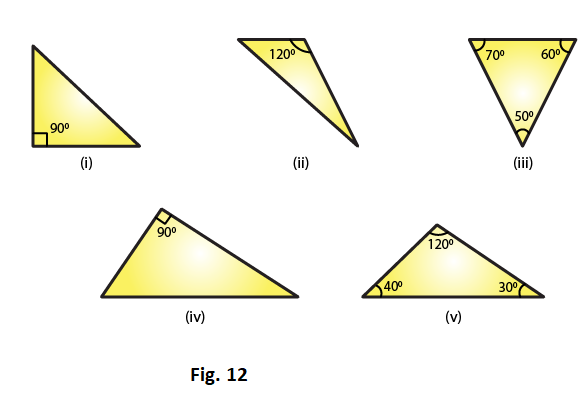Solution:
(i) The given triangle is a right triangle because one of its angles is 90o.
(ii) The given triangle is an obtuse triangle because one of its angles is 120o, which is greater than 90o
(iii) The given triangle is an acute triangle because all its angles are less than 90o
(iv) The given triangle is a right triangle because one of its angles is 90o.
(v) The given triangle is an obtuse triangle because one of its angles is 110o, which is greater than 90o.
10. Fill in the blanks with the correct word/symbol to make it a true statement:
(i) A triangle has ……. sides.
(ii) A triangle has ……..vertices.
(iii) A triangle has ……..angles.
(iv) A triangle has ………parts.
(v) A triangle whose no two sides are equal is known as ………
(vi) A triangle whose two sides are equal is known as ……….
(vii) A triangle whose all the sides are equal is known as ………
(viii) A triangle whose one angle is a right angle is known as ……..
(ix) A triangle whose all the angles are of measure less than 90′ is known as ………
(x) A triangle whose one angle is more than 90′ is known as ……….
Solution:
(i) Three
(ii) Three
(iii) Three
(iv) Six
(v) A scalene triangle
(vi) An isosceles triangle
(vii) An equilateral triangle
(viii) A right triangle
(ix) An acute triangle
(x) An obtuse triangle
11. In each of the following, state if the statement is true (T) or false (F):
(i) A triangle has three sides.
(ii) A triangle may have four vertices.
(iii) Any three line-segments make up a triangle.
(iv) The interior of a triangle includes its vertices.
(v) The triangular region includes the vertices of the corresponding triangle.
(vi) The vertices of a triangle are three collinear points.
(vii) An equilateral triangle is isosceles also.
(viii) Every right triangle is scalene.
(ix) Each acute triangle is equilateral.
(x) No isosceles triangle is obtuse.
Solution:
(i) True
(ii) False
Explanation:
Any three non-parallel line segments can make up a triangle.
(iii) False.
Explanation:
Any three non-parallel line segments can make up a triangle.
(iv) False.
Explanation:
The interior of a triangle is the region enclosed by the triangle and the vertices are not enclosed by the triangle.
(v) True.
Explanation:
The triangular region includes the interior region and the triangle itself.
(vi) False.
Explanation:
The vertices of a triangle are three non-collinear points.
(vii) True.
Explanation:
In an equilateral triangle, any two sides are equal.
(viii) False.
Explanation:
A right triangle can also be an isosceles triangle.
(ix) False.
Explanation:
Each acute triangle is not an equilateral triangle, but each equilateral triangle is an acute triangle.
(x) False.
Explanation:
An isosceles triangle can be an obtuse triangle, a right triangle or an acute triangle

Exercise 15.2 Page No: 15.12
1. Two angles of a triangle are of measures 150o and 30o. Find the measure of the third angle.
Solution:
Given two angles of a triangle are of measures 150o and 30o
Let the required third angle be x
We know that sum of all the angles of a triangle = 180o
105o + 30o + x = 180o
135o + x = 180o
x = 180o – 135o
x = 45o
Therefore the third angle is 45o
2. One of the angles of a triangle is 130o, and the other two angles are equal. What is the measure of each of these equal angles?
Solution:
Given one of the angles of a triangle is 130o
Also given that remaining two angles are equal
So let the second and third angle be x
We know that sum of all the angles of a triangle = 180o
130o + x + x = 180o
130o + 2x = 180o
2x = 180– 130o
2x = 50o
x = 50/2
x = 25o
Therefore the two other angles are 25o each
3. The three angles of a triangle are equal to one another. What is the measure of each of the angles?
Solution:
Given that three angles of a triangle are equal to one another
So let the each angle be x
We know that sum of all the angles of a triangle = 180o
x + x + x = 180o
3x = 180o
x = 180/3
x = 60o
Therefore angle is 60o each
4. If the angles of a triangle are in the ratio 1: 2: 3, determine three angles.
Solution:
Given angles of the triangle are in the ratio 1: 2: 3
So take first angle as x, second angle as 2x and third angle as 3x
We know that sum of all the angles of a triangle = 180o
x + 2x + 3x = 180o
6x = 180o
x = 180/6
x = 30o
2x = 30o × 2 = 60o
3x = 30o × 3 = 90o
Therefore the first angle is 30o, second angle is 60o and third angle is 90o.
5. The angles of a triangle are (x − 40)o, (x − 20)o and (1/2 − 10)o. Find the value of x.
Solution:
Given the angles of a triangle are (x − 40)o, (x − 20)o and (1/2 − 10)o.
We know that sum of all the angles of a triangle = 180o
(x − 40)o + (x − 20)o + (1/2 − 10)o = 180o
x + x + (x/2) – 40o – 20– 10o = 180o
x + x + (x/2) – 70o = 180o
(5x/2) = 180+ 70o
(5x/2) = 250o
x = (2/5) × 250o
x = 100o
Hence the value of x is 100o
6. The angles of a triangle are arranged in ascending order of magnitude. If the difference between two consecutive angles is 10o. Find the three angles.
Solution:
Given that angles of a triangle are arranged in ascending order of magnitude
Also given that difference between two consecutive angles is 10o
Let the first angle be x
Second angle be x + 10o
Third angle be x + 10o + 10o
We know that sum of all the angles of a triangle = 180o
x + x + 10o + x + 10o +10o = 180o
3x + 30 = 180
3x = 180 – 30
3x = 150
x = 150/3
x = 50o
First angle is 50o
Second angle x + 10o = 50 + 10 = 60o
Third angle x + 10o +10o = 50 + 10 + 10 = 70o
7. Two angles of a triangle are equal and the third angle is greater than each of those angles by 30o. Determine all the angles of the triangle
Solution:
Given that two angles of a triangle are equal
Let the first and second angle be x
Also given that third angle is greater than each of those angles by 30o
Therefore the third angle is greater than the first and second by 30o = x + 30o
The first and the second angles are equal
We know that sum of all the angles of a triangle = 180o
x + x + x + 30o = 180o
3x + 30 = 180
3x = 180 – 30
3x = 150
x = 150/3
x = 50o
Third angle = x + 30o = 50o + 30o = 80o
The first and the second angle is 50o and the third angle is 80o.
8. If one angle of a triangle is equal to the sum of the other two, show that the triangle is a right triangle.
Solution:
Given that one angle of a triangle is equal to the sum of the other two
Let the measure of angles be x, y, z
Therefore we can write above statement as x = y + z
x + y + z = 180o
Substituting the above value we get
x + x = 180o
2x = 180o
x = 180/2
x = 90o
If one angle is 90o then the given triangle is a right angled triangle
9. If each angle of a triangle is less than the sum of the other two, show that the triangle is acute angled.
Solution:
Given that each angle of a triangle is less than the sum of the other two
Let the measure of angles be x, y and z
From the above statement we can write as
x > y + z
y < x + z
z < x + y
Therefore triangle is an acute triangle
10. In each of the following, the measures of three angles are given. State in which cases the angles can possibly be those of a triangle:
(i) 63o, 37o, 80o
(ii) 45o, 61o, 73o
(iii) 59o, 72o, 61o
(iv) 45o, 45o, 90o
(v) 30o, 20o, 125o
Solution:
(i) 63o + 37+ 80o = 180o
Angles form a triangle
(ii) 45o, 61o, 73o is not equal to 180o
Therefore not a triangle
(iii) 59o, 72o, 61o is not equal to 1800
Therefore not a triangle
(iv) 45o+ 45o+ 90o = 180o
Angles form a triangle
(v) 30o, 20o, 125o is not equal to 180o
Therefore not a triangle
11. The angles of a triangle are in the ratio 3: 4: 5. Find the smallest angle
Solution:
Given that angles of a triangle are in the ratio: 3: 4: 5
Therefore let the measure of the angles be 3x, 4x, 5x
We know that sum of the angles of a triangle =180o
3x + 4x + 5x = 180o
12x = 180o
x = 180/12
x = 15o
Smallest angle = 3x
= 3 × 15o
= 45o
Therefore smallest angle = 45o
12. Two acute angles of a right triangle are equal. Find the two angles.
Solution:
Given that acute angles of a right angled triangle are equal
We know that Right triangle: whose one of the angle is a right angle
Let the measure of angle be x, x, 90o
x + x + 90o= 180o
2x = 90o
x = 90/2
x = 45o
The two angles are 45o and 45o
13. One angle of a triangle is greater than the sum of the other two. What can you say about the measure of this angle? What type of a triangle is this?
Solution:
Given one angle of a triangle is greater than the sum of the other two
Let the measure of the angles be x, y, z
From the question we can write as
x > y + z or
y > x + z   or
z > x + y
x or y or z > 90o which is obtuse
Therefore triangle is an obtuse angle
14. In the six cornered figure, (fig. 20), AC, AD and AE are joined. Find ∠FAB + ∠ABC + ∠BCD + ∠CDE + ∠DEF + ∠EFA.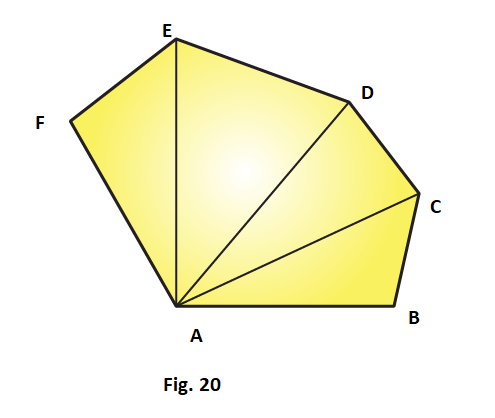Solution:
We know that sum of the angles of a triangle is 180o
Therefore in ∆ABC, we have
∠CAB + ∠ABC + ∠BCA = 180…….. (i)
In△ ACD, we have
∠DAC + ∠ACD + ∠CDA = 180…….. (ii)
In △AEF, we have
∠FAE + ∠AEF + ∠EFA = 180o ………. (iv)
Adding (i), (ii), (iii), (iv) we get
∠CAB + ∠ABC + ∠BCA + ∠DAC + ∠ACD + ∠CDA + ∠EAD + ∠ADE + ∠DEA + ∠FAE + ∠AEF +∠EFA = 720o
Therefore ∠FAB + ∠ABC + ∠BCD + ∠CDE + ∠DEF + ∠EFA = 720o
15. Find x, y, z (whichever is required) from the figures (Fig. 21) given below: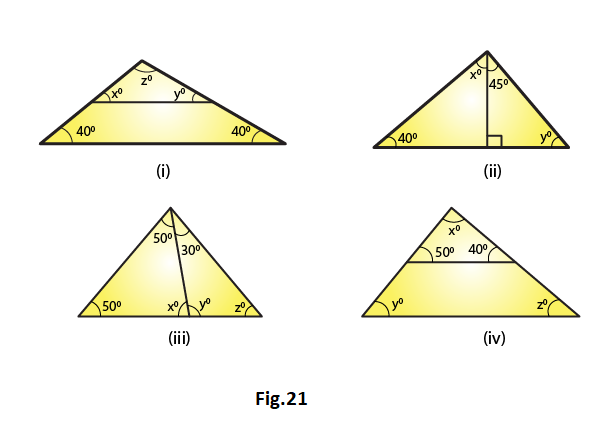Solution:
(i) In △ABC and △ADE we have,
∠ ADE = ∠ ABC [corresponding angles]
x = 40o
∠AED = ∠ACB (corresponding angles)
y = 30o
We know that the sum of all the three angles of a triangle is equal to 180o
x + y + z = 180o (Angles of △ADE)
Which means: 40o + 30o + z = 180o
z = 180o – 70o
z = 110o
Therefore, we can conclude that the three angles of the given triangle are 40o, 30o and 110o
(ii)  We can see that in △ADC, ∠ADC is equal to 90o.
We also know that the sum of all the angles of a triangle is equal to 180o.
Which means: 45o + 90o + y = 180o (Sum of the angles of △ADC)
135o + y = 180o
y = 180o – 135o.
y = 45o.
We can also say that in △ ABC, ∠ABC + ∠ACB + ∠BAC is equal to 180o.
(Sum of the angles of △ABC)
40o + y + (x + 45o) = 180o
40o + 45o + x + 45o = 180o (y = 45o)
x = 180o –130o
x = 50o
Therefore, we can say that the required angles are 45o and 50o.
(iii) We know that the sum of all the angles of a triangle is equal to 180o.
Therefore, for △ABD:
∠ABD +∠ADB + ∠BAD = 180° (Sum of the angles of △ABD)
50o + x + 50o = 180o
100o + x = 180o
x = 180o – 100o
x = 80o
For △ ABC:
∠ABC + ∠ACB + ∠BAC = 180o (Sum of the angles of △ABC)
50o + z + (50o + 30o) = 180o
50o + z + 50o + 30o = 180o
z = 180o – 130o
z = 50o
Using the same argument for △ADC:
∠ADC + ∠ACD + ∠DAC = 180o (Sum of the angles of △ADC)
y + z + 30o = 180o
y + 50o + 30o = 180o (z = 50o)
y = 180o – 80o
y = 1000
Therefore, we can conclude that the required angles are 80o, 50o and 100o.
(iv) In △ABC and △ADE we have:
y = 50o
Also, ∠AED = ∠ACB (Corresponding angles)
z = 40o
We know that the sum of all the three angles of a triangle is equal to 180o.
We can write as x + 50o + 40o = 180o (Angles of △ADE)
x = 180o – 90o
x = 90o
Therefore, we can conclude that the required angles are 50o, 40o and 90o.
16. If one angle of a triangle is 60o and the other two angles are in the ratio 1: 2, find the angles.
Solution:
Given that one of the angles of the given triangle is 60o.
Also given that the other two angles of the triangle are in the ratio 1: 2.
Let one of the other two angles be x.
Therefore, the second one will be 2x.
We know that the sum of all the three angles of a triangle is equal to 180o.
60o + x + 2x = 180o
3x = 180o – 60o
3x = 120o
x = 120o/3
x = 40o
2x = 2 × 40o
2x = 80o
Hence, we can conclude that the required angles are 40o and 80o.
17. It one angle of a triangle is 100o and the other two angles are in the ratio 2: 3. Find the angles.
Solution:
Given that one of the angles of the given triangle is 100o.
Also given that the other two angles are in the ratio 2: 3.
Let one of the other two angles be 2x.
Therefore, the second angle will be 3x.
We know that the sum of all three angles of a triangle is 180o.
100o + 2x + 3x = 180o
5x = 180o – 100o
5x = 80o
x = 80/5
x = 16
2x = 2 ×16
2x = 32o
3x = 3×16
3x = 48o
Thus, the required angles are 32o and 48o.

18. In △ABC, if 3∠A = 4∠B = 6∠C, calculate the angles.
Solution:
We know that for the given triangle, 3∠A = 6∠C
∠A = 2∠C ……. (i)
We also know that for the same triangle, 4∠B = 6∠C
∠B = (6/4) ∠C …… (ii)
We know that the sum of all three angles of a triangle is 180o.
Therefore, we can say that:
∠A + ∠B + ∠C = 180o (Angles of △ABC)…… (iii)
On putting the values of ∠A and ∠B in equation (iii), we get:
2∠C + (6/4) ∠C + ∠C = 180o
(18/4) ∠C = 180o
∠C = 40o
From equation (i), we have:
∠A = 2∠C = 2 × 40
∠A = 80o
From equation (ii), we have:
∠B = (6/4) ∠C = (6/4) × 40o
∠B = 60o
∠A = 80o, ∠B = 60o, ∠C = 40o
Therefore, the three angles of the given triangle are 80o, 60o, and 40o.
19. Is it possible to have a triangle, in which
(i) Two of the angles are right?
(ii) Two of the angles are obtuse?
(iii) Two of the angles are acute?
(iv) Each angle is less than 60o?
(v) Each angle is greater than 60o?
(vi) Each angle is equal to 60o?
Solution:
(i) No, because if there are two right angles in a triangle, then the third angle of the triangle must be zero, which is not possible.
(ii) No, because as we know that the sum of all three angles of a triangle is always 180o. If there are two obtuse angles, then their sum will be more than 180o, which is not possible in case of a triangle.
(iii) Yes, in right triangles and acute triangles, it is possible to have two acute angles.
(iv) No, because if each angle is less than 60o, then the sum of all three angles will be less than 180o, which is not possible in case of a triangle.
20. In△ ABC, ∠A = 100o, AD bisects ∠A and AD ⊥ BC. Find ∠B
Solution:Given that in △ABC, ∠A = 100o
Also given that AD ⊥ BC
Consider △ABD
We know that the sum of all three angles of a triangle is 180o.
Thus,
∠ABD + ∠BAD + ∠ADB = 180o (Sum of angles of △ABD)
Or,
∠ABD + 50o + 90o = 180o
∠ABD =180o – 140o
∠ABD = 40o
21. In △ABC, ∠A = 50o, ∠B = 100o and bisector of ∠C meets AB in D. Find the angles of the triangles ADC and BDC
Solution: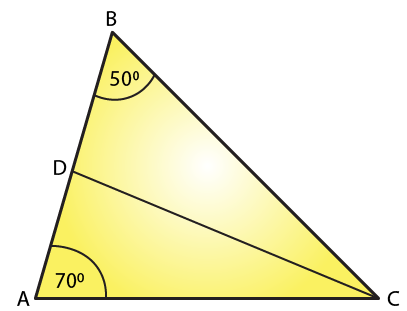We know that the sum of all three angles of a triangle is equal to 180o.
Therefore, for the given △ABC, we can say that:
∠A + ∠B + ∠C = 180o (Sum of angles of △ABC)
50o + 70o + ∠C = 180o
∠C= 180o –120o
∠C = 60o
∠ACD = ∠BCD =∠C2 (CD bisects ∠C and meets AB in D.)
∠ACD = ∠BCD = 60/2= 30o
Using the same logic for the given △ACD, we can say that:
∠DAC + ∠ACD + ∠ADC = 180o
50o + 30o + ∠ADC = 180o
If we use the same logic for the given △BCD, we can say that
∠DBC + ∠BCD + ∠BDC = 180o
70o + 30o + ∠BDC = 180o
∠BDC = 180o – 100o
∠BDC = 80o
Thus,
For △ADC: ∠A = 50o, ∠D = 100o ∠C = 30o
△BDC: ∠B = 70o, ∠D = 80o ∠C = 30o
22. In ∆ABC, ∠A = 60o, ∠B = 80o, and the bisectors of ∠B and ∠C, meet at O. Find
(i) ∠C
(ii) ∠BOC
Solution: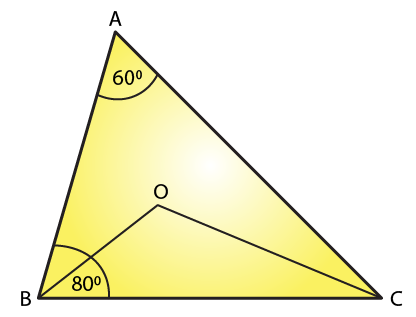(i) We know that the sum of all three angles of a triangle is 180o.
Hence, for △ABC, we can say that:
∠A + ∠B + ∠C = 180o (Sum of angles of ∆ABC)
60o + 80o + ∠C= 180o.
∠C = 180o – 140o
∠C = 140o.
(ii)For △OBC,
∠OBC = ∠B/2 = 80/2 (OB bisects ∠B)
∠OBC = 40o
∠OCB =∠C/2 = 40/2 (OC bisects ∠C)
∠OCB = 20o
If we apply the above logic to this triangle, we can say that:
∠OCB + ∠OBC + ∠BOC = 180o (Sum of angles of △OBC)
20o + 40o + ∠BOC = 180o
∠BOC = 180o – 60o
∠BOC = 120o
23. The bisectors of the acute angles of a right triangle meet at O. Find the angle at O between the two bisectors.
Solution: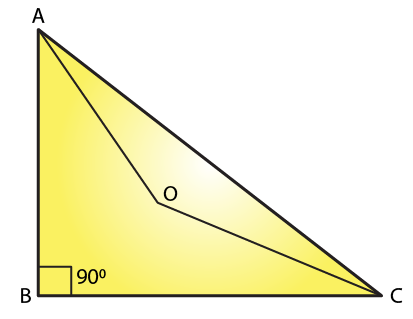Given bisectors of the acute angles of a right triangle meet at O
We know that the sum of all three angles of a triangle is 180o.
Hence, for △ABC, we can say that:
∠A + ∠B + ∠C = 180o
∠A + 90o + ∠C = 180o
∠A + ∠C = 180o – 90o
∠A + ∠C = 90o
For △OAC:
∠OAC = ∠A/2 (OA bisects LA)
∠OCA   = ∠C/2 (OC bisects LC)
On applying the above logic to △OAC, we get
∠AOC + ∠OAC + ∠OCA = 180o (Sum of angles of △AOC)
∠AOC + ∠A2 + ∠C2 = 180o
∠AOC + ∠A + ∠C2 = 180o
∠AOC + 90/2 = 180o
∠AOC = 180o – 45o
∠AOC = 135o
24.  In △ABC, ∠A = 50o and BC is produced to a point D. The bisectors of ∠ABC and ∠ACD meet at E. Find ∠E.
Solution: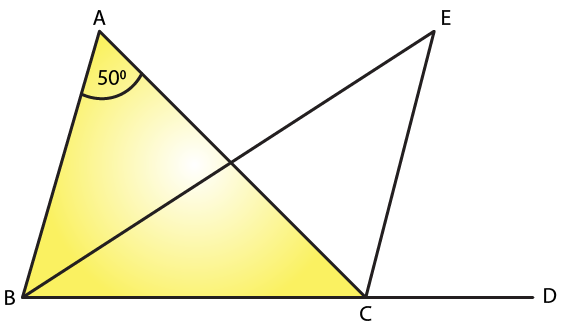In the given triangle,
∠ACD = ∠A + ∠B. (Exterior angle is equal to the sum of two opposite interior angles.)
We know that the sum of all three angles of a triangle is 180o.
Therefore, for the given triangle, we know that the sum of the angles = 180o
∠ABC + ∠BCA + ∠CAB = 180o
∠A + ∠B + ∠BCA = 180o
∠BCA = 180o – (∠A + ∠B)
But we know that EC bisects ∠ACD
Therefore ∠ECA = ∠ACD/2
∠ECA = (∠A + ∠B)/2 [∠ACD = (∠A + ∠B)]
But EB bisects ∠ABC
∠EBC = ∠ABC/2 = ∠B/2
∠EBC = ∠ECA + ∠BCA
∠EBC = (∠A + ∠B)/2 + 180o – (∠A + ∠B)
If we use same steps for △EBC, then we get,
∠B/2 + (∠A + ∠B)/2 + 180o – (∠A + ∠B) + ∠BEC = 180o
∠BEC = ∠A + ∠B – (∠A + ∠B)/2 – ∠B/2
∠BEC = ∠A/2
∠BEC = 50o/2
= 25o
25. In ∆ABC, ∠B = 60°, ∠C = 40°, AL ⊥ BC and AD bisects ∠A such that L and D lie on side BC. Find ∠LAD
Solution: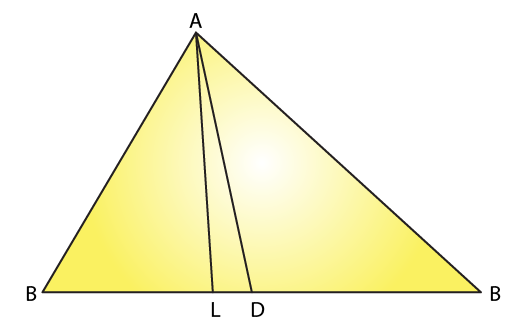We know that the sum of all angles of a triangle is 180°
Consider △ABC, we can write as
∠A + ∠B + ∠C = 180o
∠A + 60o + 40o = 180o
∠A = 800
But we know that ∠DAC bisects ∠A
∠DAC = ∠A/2
∠DAC = 80o/2
If we apply same steps for the △ADC, we get
We know that the sum of all angles of a triangle is 180°
∠ADC + ∠DCA + ∠DAC = 180o
∠ADC + 40o + 40o = 180o
We know that exterior angle is equal to the sum of two interior opposite angles
Therefore we have
But here AL perpendicular to BC
26. Line segments AB and CD intersect at O such that AC ∥ DB. It ∠CAB = 35o and ∠CDB = 55o. Find ∠BOD.
Solution: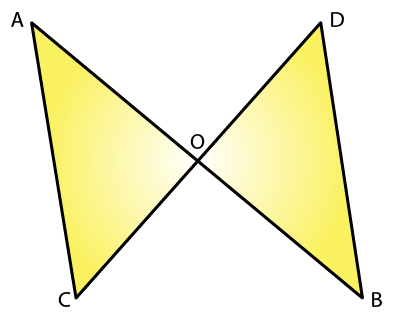We know that AC parallel to BD and AB cuts AC and BD at A and B, respectively.
∠CAB = ∠DBA (Alternate interior angles)
∠DBA = 35o
We also know that the sum of all three angles of a triangle is 180o.
Hence, for △OBD, we can say that:
∠DBO + ∠ODB + ∠BOD = 180°
35o + 55o + ∠BOD = 180o (∠DBO = ∠DBA and ∠ODB = ∠CDB)
∠BOD = 180o – 90o
∠BOD = 90o
27. In Fig. 22, ∆ABC is right angled at A, Q and R are points on line BC and P is a point such that QP ∥ AC and RP ∥ AB. Find ∠P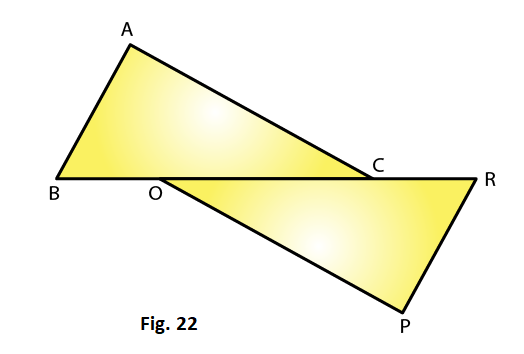Solution:
In the given triangle, AC parallel to QP and BR cuts AC and QP at C and Q, respectively.
∠QCA = ∠CQP (Alternate interior angles)
Because RP parallel to AB and BR cuts AB and RP at B and R, respectively,
∠ABC = ∠PRQ (alternate interior angles).
We know that the sum of all three angles of a triangle is 180o.
Hence, for ∆ABC, we can say that:
∠ABC + ∠ACB + ∠BAC = 180o
∠ABC + ∠ACB + 90o = 180o (Right angled at A)
∠ABC + ∠ACB = 90o
Using the same logic for △PQR, we can say that:
∠PQR + ∠PRQ + ∠QPR = 180o
∠ABC + ∠ACB + ∠QPR = 180o (∠ABC = ∠PRQ and ∠QCA = ∠CQP)
Or,
90o+ ∠QPR =180o (∠ABC+ ∠ACB = 90o)
∠QPR = 90o

Exercise 15.3 Page No: 15.19
1. In Fig. 35, ∠CBX is an exterior angle of ∆ABC at B. Name
(ii) The interior opposite angles to exterior ∠CBX
Also, name the interior opposite angles to an exterior angle at A.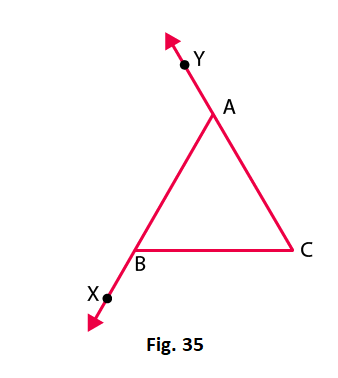Solution:
(i) The interior adjacent angle is ∠ABC
(ii) The interior opposite angles to exterior ∠CBX is ∠BAC and ∠ACB
Also the interior angles opposite to exterior are ∠ABC and ∠ACB
2. In the fig. 36, two of the angles are indicated. What are the measures of ∠ACX and ∠ACB?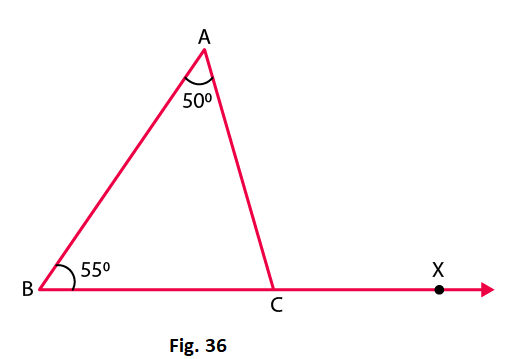Solution:
Given that in △ABC, ∠A = 50o and ∠B = 55o
We know that the sum of angles in a triangle is 180o
Therefore we have
∠A + ∠B + ∠C = 180o
50o+ 55o+ ∠C = 180o
∠C = 75o
∠ACB = 75o
∠ACX = 180o− ∠ACB = 180− 75o = 105o
3. In a triangle, an exterior angle at a vertex is 95o and its one of the interior opposite angles is 55o. Find all the angles of the triangle.
Solution: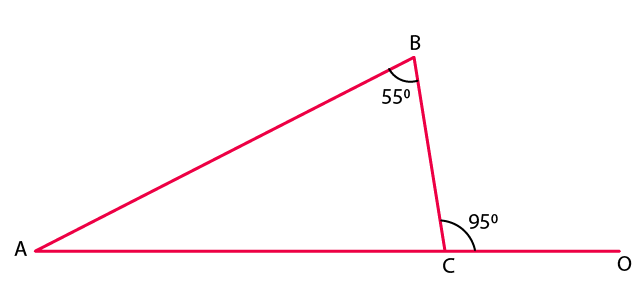We know that the sum of interior opposite angles is equal to the exterior angle.
Hence, for the given triangle, we can say that:
∠ABC+ ∠BAC = ∠BCO
55o + ∠BAC = 95o
∠BAC= 95o– 95o
∠BAC = 40o
We also know that the sum of all angles of a triangle is 180o.
Hence, for the given △ABC, we can say that:
∠ABC + ∠BAC + ∠BCA = 180o
55o + 40o + ∠BCA = 180o
∠BCA = 180o –95o
∠BCA = 85o
4. One of the exterior angles of a triangle is 80o, and the interior opposite angles are equal to each other. What is the measure of each of these two angles?
Solution:
Let us assume that A and B are the two interior opposite angles.
We know that ∠A is equal to ∠B.
We also know that the sum of interior opposite angles is equal to the exterior angle.
Therefore from the figure we have,
∠A + ∠B = 80o
∠A +∠A = 80o (because ∠A = ∠B)
2∠A = 80o
∠A = 40/2 =40o
∠A= ∠B = 40o
Thus, each of the required angles is of 40o.
5. The exterior angles, obtained on producing the base of a triangle both ways are 104o and 136o. Find all the angles of the triangle.
Solution: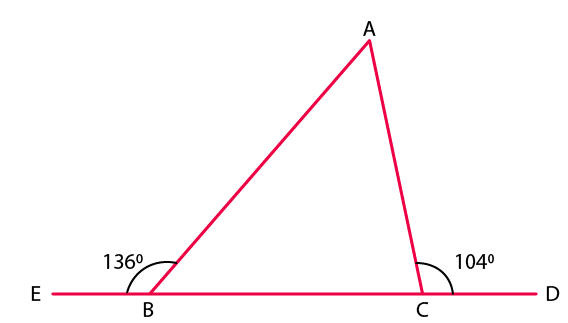In the given figure, ∠ABE and ∠ABC form a linear pair.
∠ABE + ∠ABC =180o
∠ABC = 180o– 136o
∠ABC = 44o
We can also see that ∠ACD and ∠ACB form a linear pair.
∠ACD + ∠ACB = 180o
∠AUB = 180o– 104o
∠ACB = 76o
We know that the sum of interior opposite angles is equal to the exterior angle.
Therefore, we can write as
∠BAC + ∠ABC = 104o
∠BAC = 104o – 44o = 60o
Thus,
∠ACE = 76o and ∠BAC = 60o
6. In Fig. 37, the sides BC, CA and BA of a △ABC have been produced to D, E and F respectively. If ∠ACD = 105o and ∠EAF = 45o; find all the angles of the △ABC.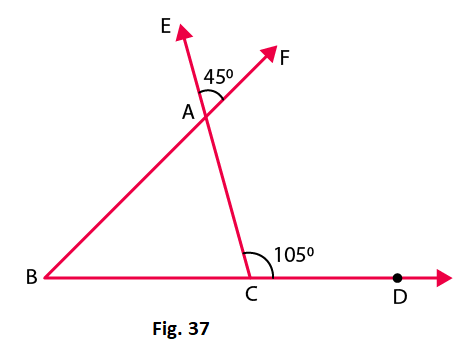Solution:
In a △ABC, ∠BAC and ∠EAF are vertically opposite angles.
Hence, we can write as
∠BAC = ∠EAF = 45o
Considering the exterior angle property, we have
∠BAC + ∠ABC = ∠ACD = 105o
On rearranging we get
∠ABC = 105o– 45o = 60o
We know that the sum of angles in a triangle is 180o
∠ABC + ∠ACS +∠BAC = 180°
∠ACB = 75o
Therefore, the angles are 45o, 65o and 75o.
7. In Fig. 38, AC perpendicular to CE and C ∠A: ∠B: ∠C= 3: 2: 1. Find the value of ∠ECD.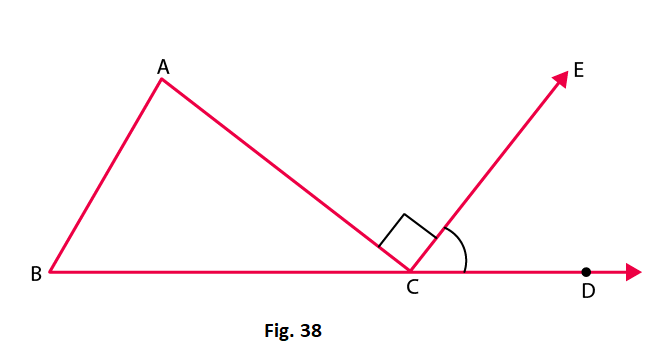Solution:
In the given triangle, the angles are in the ratio 3: 2: 1.
Let the angles of the triangle be 3x, 2x and x.
We know that sum of angles in a triangle is 180o
3x + 2x + x = 180o
6x = 180o
x = 30o
Also, ∠ACB + ∠ACE + ∠ECD = 180o
x + 90o + ∠ECD = 180o (∠ACE = 90o)
We know that x = 30o
Therefore
∠ECD = 60o
8. A student when asked to measure two exterior angles of △ABC observed that the exterior angles at A and B are of 103o and 74o respectively. Is this possible? Why or why not?
Solution:
We know that sum of internal and external angle is equal to 180o
Internal angle at A + External angle at A = 180o
Internal angle at A + 103o =180o
Internal angle at A = 77o
Internal angle at B + External angle at B = 180o
Internal angle at B + 74o = 180o
Internal angle at B = 106o
Sum of internal angles at A and B = 77o + 106o = 183o
It means that the sum of internal angles at A and B is greater than 180o, which cannot be possible.
9. In Fig.39, AD and CF are respectively perpendiculars to sides BC and AB of △ABC. If ∠FCD = 50o, find ∠BAD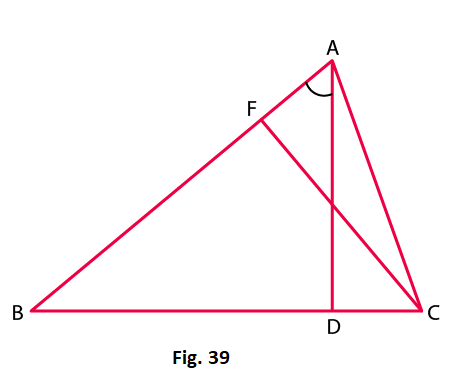Solution:
We know that the sum of all angles of a triangle is 180o
Therefore, for the given △FCB, we have
∠FCB + ∠CBF + ∠BFC = 180o
50o + ∠CBF + 90= 180o
∠CBF = 180o – 50o – 90o = 40o
Using the above steps for △ABD, we can say that:
∠ABD + ∠BDA + ∠BAD = 180o
∠BAD = 180o – 90o – 40o = 50o
10. In Fig.40, measures of some angles are indicated. Find the value of x.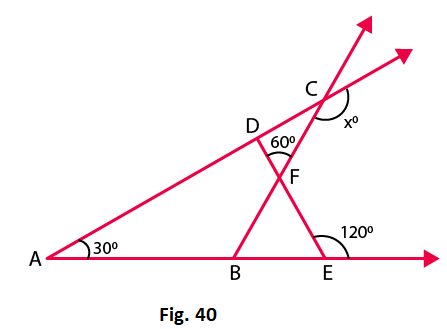Solution:
We know that the sum of the angles of a triangle is 180o
From the figure we have,
∠AED + 120o = 180o (Linear pair)
∠AED = 180o – 120o = 60o
We know that the sum of all angles of a triangle is 180o.
∠ADE + ∠AED + ∠DAE = 180o
∠ADE = 180– 60– 30o = 90o
From the given figure, we have
∠FDC + 90o = 180o (Linear pair)
∠FDC = 180– 90o = 90o
Using the same steps for △CDF, we get
∠CDF + ∠DCF + ∠DFC = 180o
90o + ∠DCF + 60o = 180o
∠DCF = 180– 60– 90= 30o
Again from the figure we have
∠DCF + x = 180o (Linear pair)
30o + x = 180o
x = 180o – 30o = 150o
11. In Fig. 41, ABC is a right triangle right angled at A. D lies on BA produced and DE perpendicular to BC intersecting AC at F. If ∠AFE = 130o, find
(i) ∠BDE
(ii) ∠BCA
(iii) ∠ABC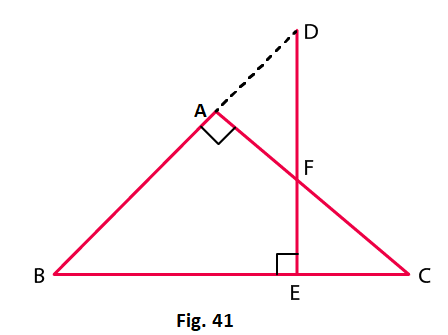Solution:
(i) Here,
∠BAF + ∠FAD = 180o (Linear pair)
∠FAD = 180– ∠BAF = 180– 90o = 90o
Also from the figure,
∠ADF = 130o − 90o = 40o
(ii) We know that the sum of all the angles of a triangle is 180o.
Therefore, for △BDE, we have
∠BDE + ∠BED + ∠DBE = 180o
∠DBE = 180– ∠BDE
∠BED = 180– 90– 40= 50o …. Equation (i)
Again from the figure we have,
∠FAD = ∠ABC + ∠ACB (Exterior angle property)
90o = 50o + ∠ACB
∠ACB = 90– 50o = 40o
(iii) From equation we have
∠ABC = ∠DBE = 50o
12. ABC is a triangle in which ∠B = ∠C and ray AX bisects the exterior angle DAC. If ∠DAX = 70o. Find ∠ACB.
Solution: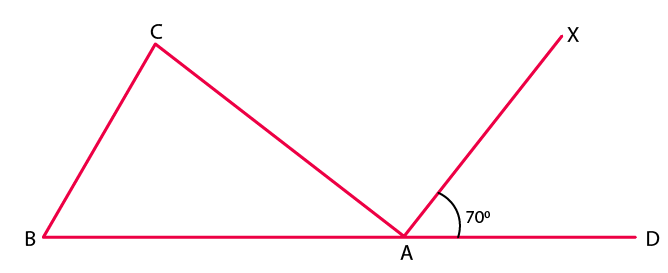Given that ABC is a triangle in which ∠B = ∠C
Also given that AX bisects the exterior angle DAC
∠CAX = ∠DAX (AX bisects ∠CAD)
∠CAX =70[given]
∠CAX +∠DAX + ∠CAB =180o
70o+ 70o + ∠CAB =180o
∠CAB =180o –140o
∠CAB =40o
∠ACB + ∠CBA + ∠CAB = 180o (Sum of the angles of ∆ABC)
∠ACB + ∠ACB+ 40o = 180o (∠C = ∠B)
2∠ACB = 180– 40o
∠ACB = 140/2
∠ACB = 70o
13. The side BC of △ABC is produced to a point D. The bisector of ∠A meets side BC in L. If ∠ABC= 30o and ∠ACD = 115o, find ∠ALC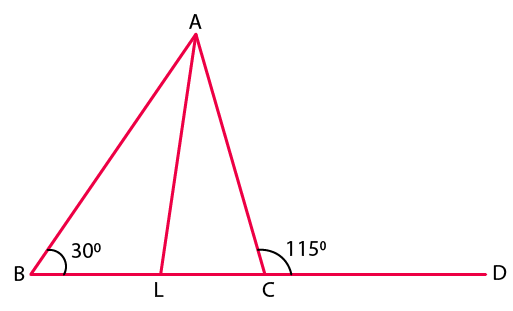Solution:
Given that ∠ABC= 30o and ∠ACD = 115o
From the figure, we have
∠ACD and ∠ACL make a linear pair.
∠ACD+ ∠ACB = 180o
115o + ∠ACB =180o
∠ACB = 180– 115o
∠ACB = 65o
We know that the sum of all angles of a triangle is 180o.
Therefore, for△ ABC, we have
∠ABC + ∠BAC + ∠ACB = 180o
30o + ∠BAC + 65o = 180o
∠BAC = 85o
∠LAC = ∠BAC/2 = 85/2
Using the same steps for △ALC, we get
∠ALC + ∠LAC + ∠ACL = 180o
∠ALC + 82/2 + 65o = 180o
We know that ∠ALC = ∠ACB
∠ALC = 180o – 82/2 – 65o
∠ALC = 72 ½o
14. D is a point on the side BC of △ABC. A line PDQ through D, meets side AC in P and AB produced at Q. If ∠A = 80o, ∠ABC = 60o and ∠PDC = 15o, find
(i) ∠AQD
(ii) ∠APD
Solution: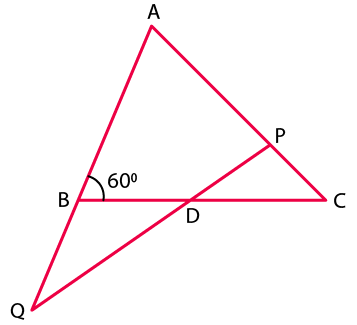From the figure we have
∠ABD and ∠QBD form a linear pair.
∠ABC + ∠QBC =180o
60o + ∠QBC = 180o
∠QBC = 120o
∠PDC = ∠BDQ (Vertically opposite angles)
∠BDQ = 75o
In △QBD:
∠QBD + ∠QDB + ∠BDQ = 180o (Sum of angles of △QBD)
120o+ 15o + ∠BQD = 180o
∠BQD = 180– 135o
∠BQD = 45o
∠AQD = ∠BQD = 45o
In △AQP:
∠QAP + ∠AQP + ∠APQ = 180o (Sum of angles of △AQP)
80o + 45o + ∠APQ = 180o
∠APQ = 55o
∠APD = ∠APQ
15. Explain the concept of interior and exterior angles and in each of the figures given below. Find x and y (Fig. 42)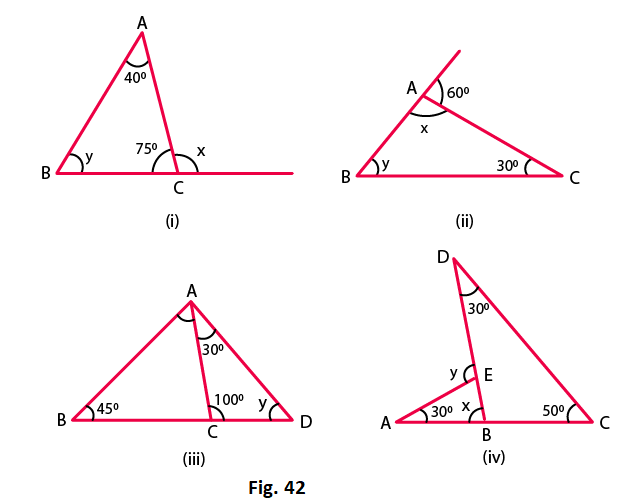Solution:
The interior angles of a triangle are the three angle elements inside the triangle.
The exterior angles are formed by extending the sides of a triangle, and if the side of a triangle is produced, the exterior angle so formed is equal to the sum of the two interior opposite angles.
Using these definitions, we will obtain the values of x and y.
(i) From the given figure, we have
∠ACB + x = 180o (Linear pair)
75o+ x = 180o
x = 105o
We know that the sum of all angles of a triangle is 180o
Therefore, for △ABC, we can say that:
∠BAC+ ∠ABC +∠ACB = 180o
40o+ y +75o = 180o
y = 65o
(ii) From the figure, we have
x + 80= 180o (Linear pair)
x = 100o
In △ABC, we have
We also know that the sum of angles of a triangle is 180o
x + y + 30o = 180o
100o + 30o + y = 180o
y = 50o
(iii) We know that the sum of all angles of a triangle is 180°.
Therefore, for △ACD, we have
30o + 100o + y = 180o
y = 50o
Again from the figure we can write as
∠ACB + 100° = 180°
∠ACB = 80o
Using the above rule for △ACD, we can say that:
x + 45o + 80o = 180o
x = 55o
(iv) We know that the sum of all angles of a triangle is 180o.
Therefore, for △DBC, we have
30o + 50o + ∠DBC = 180o
∠DBC = 100o
From the figure we can say that
x + ∠DBC = 180o is a Linear pair
x = 80o
From the exterior angle property we have
y = 30o + 80o = 110o
16. Compute the value of x in each of the following figures: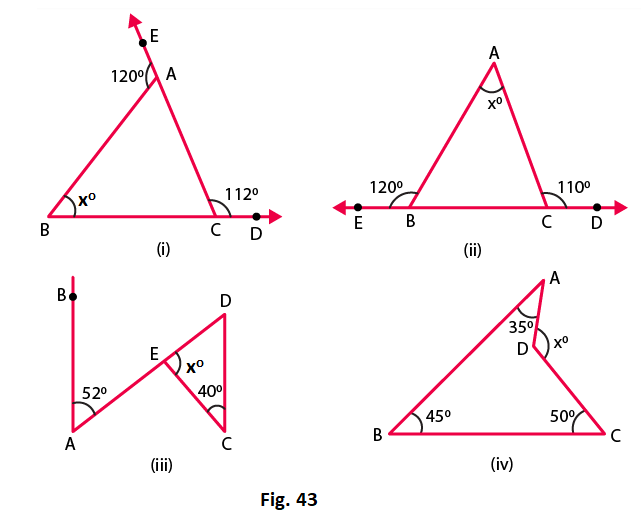Solution:
(i) From the given figure, we can write as
∠ACD + ∠ACB = 180o is a linear pair
On rearranging we get
∠ACB = 180– 112o = 68o
Again from the figure we have,
∠BAE + ∠BAC = 180o is a linear pair
On rearranging we get,
∠BAC = 180o– 120o = 60o
We know that the sum of all angles of a triangle is 180o.
Therefore, for △ABC:
x + ∠BAC + ∠ACB = 180o
x = 180– 60– 68o = 52o
x = 52o
(ii) From the given figure, we can write as
∠ABC + 120o = 180o is a linear pair
∠ABC = 60o
Again from the figure we can write as
∠ACB+ 110o = 180o is a linear pair
∠ACB = 70o
We know that the sum of all angles of a triangle is 180o.
Therefore, consider △ABC, we get
x + ∠ABC + ∠ACB = 180o
x = 50o
(iii) From the given figure, we can write as
We know that the sum of all the angles of a triangle is 180o.
Therefore, consider △DEC, we have
x + 40+ 52= 180o
x = 88o
(iv) In the given figure, we have a quadrilateral and also we know that sum of all angles is quadrilateral is 360o.
Thus,
35o + 45o + 50o + reflex ∠ADC = 360o
On rearranging we get,
230o + x = 360o (A complete angle)
x = 130o

Exercise 15.4 Page No: 15.24
1. In each of the following, there are three positive numbers. State if these numbers could possibly be the lengths of the sides of a triangle:
(i) 5, 7, 9
(ii) 2, 10, 15
(iii) 3, 4, 5
(iv) 2, 5, 7
(v) 5, 8, 20
Solution:
(i) Given 5, 7, 9
Yes, these numbers can be the lengths of the sides of a triangle because the sum of any two sides of a triangle is always greater than the third side.
Here, 5 + 7 > 9, 5 + 9 > 7, 9 + 7 > 5
(ii) Given 2, 10, 15
No, these numbers cannot be the lengths of the sides of a triangle because the sum of any two sides of a triangle is always greater than the third side, which is not true in this case.
(iii) Given 3, 4, 5
Yes, these numbers can be the lengths of the sides of a triangle because the sum of any two sides of triangle is always greater than the third side.
Here, 3 + 4 > 5, 3 + 5 > 4, 4 + 5 > 3
(iv) Given 2, 5, 7
No, these numbers cannot be the lengths of the sides of a triangle because the sum of any two sides of a triangle is always greater than the third side, which is not true in this case.
Here, 2 + 5 = 7
(v) Given 5, 8, 20
No, these numbers cannot be the lengths of the sides of a triangle because the sum of any two sides of a triangle is always greater than the third side, which is not true in this case.
Here, 5 + 8 < 20
2. In Fig. 46, P is the point on the side BC. Complete each of the following statements using symbol ‘=’,’ > ‘or ‘< ‘so as to make it true:
(i) AP… AB+ BP
(ii) AP… AC + PC
(iii) AP…. ½ (AB + AC + BC)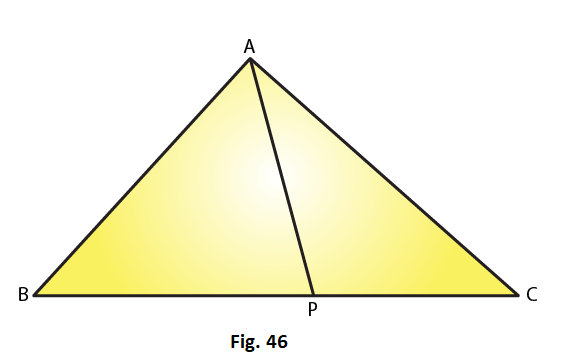Solution:
(i) In △APB, AP < AB + BP because the sum of any two sides of a triangle is greater than the third side.
(ii) In △APC, AP < AC + PC because the sum of any two sides of a triangle is greater than the third side.
(iii) AP < ½ (AB + AC + BC)
In △ABP and △ACP, we can write as
AP < AB + BP… (i) (Because the sum of any two sides of a triangle is greater than the third side)
AP < AC + PC … (ii) (Because the sum of any two sides of a triangle is greater than the third side)
On adding (i) and (ii), we have:
AP + AP < AB + BP + AC + PC
2AP < AB + AC + BC (BC = BP + PC)
AP < (AB – AC + BC)

3. P is a point in the interior of △ABC as shown in Fig. 47. State which of the following statements are true (T) or false (F):
(i) AP + PB < AB
(ii) AP + PC > AC
(iii) BP + PC = BC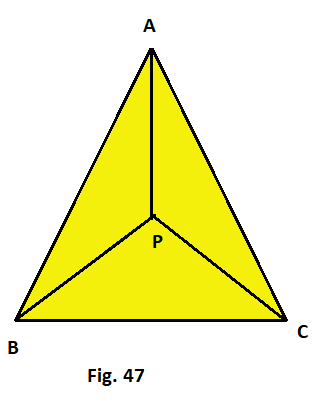Solution:
(i) False
Explanation:
We know that the sum of any two sides of a triangle is greater than the third side, it is not true for the given triangle.
(ii) True
Explanation:
We know that the sum of any two sides of a triangle is greater than the third side, it is true for the given triangle.
(iii) False
Explanation:
We know that the sum of any two sides of a triangle is greater than the third side, it is not true for the given triangle.
4. O is a point in the exterior of △ABC. What symbol ‘>’,’<’ or ‘=’ will you see to complete the statement OA+OB….AB? Write two other similar statements and show that OA + OB + OC > ½ (AB + BC +CA)
Solution:
We know that the sum of any two sides of a triangle is always greater than the third side, in △OAB, we have,
OA + OB > AB ….. (i)
OB + OC > BC …… (ii)
OA + OC > CA ….. (iii)
On adding equations (i), (ii) and (iii) we get:
OA + OB + OB + OC + OA + OC > AB + BC + CA
2(OA + OB + OC) > AB + BC + CA
OA + OB + OC > (AB + BC + CA)/2
Or
OA + OB + OC > ½ (AB + BC +CA)
Hence the proof.
5. In △ABC, ∠A = 100o, ∠B = 30o, ∠C = 50o. Name the smallest and the largest sides of the triangle.
Solution:
We know that the smallest side is always opposite to the smallest angle, which in this case is 30°, it is AC.
Also, because the largest side is always opposite to the largest angle, which in this case is 100°, it is BC.

Exercise 15.5 Page No: 15.30
1. State Pythagoras theorem and its converse.
Solution:
The Pythagoras Theorem:
In a right triangle, the square of the hypotenuse is always equal to the sum of the squares of the other two sides.
Converse of the Pythagoras Theorem:
If the square of one side of a triangle is equal to the sum of the squares of the other two sides, then the triangle is a right triangle, with the angle opposite to the first side as right angle.
2. In right △ABC, the lengths of the legs are given. Find the length of the hypotenuse
(i) a = 6 cm, b = 8 cm
(ii) a = 8 cm, b = 15 cm
(iii) a = 3 cm, b = 4 cm
(iv) a = 2 cm, b =1.5 cm
Solution:
(i) According to the Pythagoras theorem, we have
(Hypotenuse)2 = (Base)2 + (Height)2
Let c be hypotenuse and a and b be other two legs of right angled triangle
Then we have
c= a2 + b2
c2 = 62 + 82
c2 = 36 + 64 = 100
c = 10 cm
(ii) According to the Pythagoras theorem, we have
(Hypotenuse)2 = (Base)2 + (Height)2
Let c be hypotenuse and a and b be other two legs of right angled triangle
Then we have
c2 = a2 + b2
c2 = 82 + 152
c2 = 64 + 225 = 289
c = 17cm
(iii) According to the Pythagoras theorem, we have
(Hypotenuse)2 = (Base)2 + (Height)2
Let c be hypotenuse and a and b be other two legs of right angled triangle
Then we have
c2 = a2 + b2
c2 = 32 + 42
c= 9 + 16 = 25
c = 5 cm
(iv) According to the Pythagoras theorem, we have
(Hypotenuse)2 = (Base)2 + (Height)2
Let c be hypotenuse and a and b be other two legs of right angled triangle
Then we have
c= a2 + b2
c= 2+ 1.52
c2 = 4 + 2.25 = 6.25
c = 2.5 cm
3. The hypotenuse of a triangle is 2.5 cm. If one of the sides is 1.5 cm. find the length of the other side.
Solution:
Let c be hypotenuse and the other two sides be b and a
According to the Pythagoras theorem, we have
c2 = a2 + b2
2.52 = 1.52 + b2
b2 = 6.25 −2.25 = 4
b = 2 cm
Hence, the length of the other side is 2 cm.
4. A ladder 3.7 m long is placed against a wall in such a way that the foot of the ladder is 1.2 m away from the wall. Find the height of the wall to which the ladder reaches.
Solution: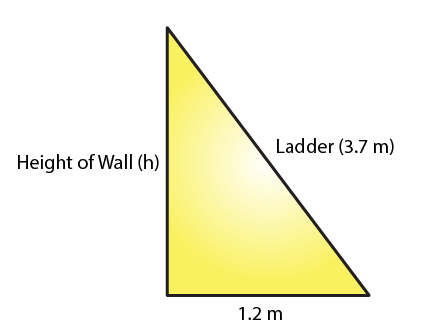Let the height of the ladder reaches to the wall be h.
According to the Pythagoras theorem, we have
(Hypotenuse)2 = (Base)2 + (Height)2
3.72 = 1.22 + h2
h2 = 13.69 – 1.44 = 12.25
h = 3.5 m
Hence, the height of the wall is 3.5 m.
5. If the sides of a triangle are 3 cm, 4 cm and 6 cm long, determine whether the triangle is right-angled triangle.
Solution:
In the given triangle, the largest side is 6 cm.
We know that in a right angled triangle, the sum of the squares of the smaller sides should be equal to the square of the largest side.
Therefore,
32 + 42 = 9 + 16 = 25
But, 62 = 36
32 + 42 = 25 which is not equal to 62
Hence, the given triangle is not a right angled triangle.
6. The sides of certain triangles are given below. Determine which of them are right triangles.
(i) a = 7 cm, b = 24 cm and c= 25 cm
(ii) a = 9 cm, b = 16 cm and c = 18 cm
Solution:
(i) We know that in a right angled triangle, the square of the largest side is equal to the sum of the squares of the smaller sides.
Here, the larger side is c, which is 25 cm.
c2 = 625
Given that,
a2+ b= 72 + 242
= 49 + 576
= 625
= c2
Thus, the given triangle is a right triangle.
(ii) We know that in a right angled triangle, the square of the largest side is equal to the sum of the squares of the smaller sides.
Here, the larger side is c, which is 18 cm.
c= 324
Given that
a2+ b= 9+ 16
= 81 + 256
= 337 which is not equal to c2
Thus, the given triangle is not a right triangle.
7. Two poles of heights 6 m and 11 m stand on a plane ground. If the distance between their feet is 12 m.  Find the distance between their tops.
(Hint: Find the hypotenuse of a right triangle having the sides (11 – 6) m = 5 m and 12 m)
Solution: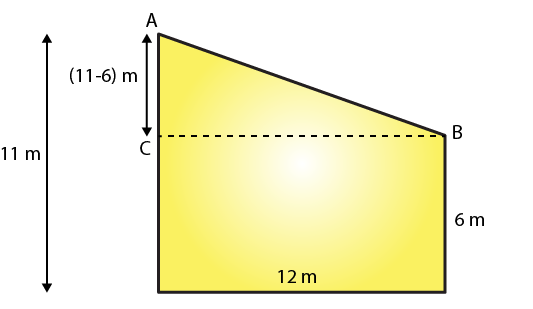Let the distance between the tops of the poles is the distance between points A and B.
We can see from the given figure that points A, B and C form a right triangle, with AB as the hypotenuse.
By using the Pythagoras Theorem in △ABC, we get
(11−6)+ 12= AB2
AB2 = 25 + 144
AB2 = 169
AB = 13
Hence, the distance between the tops of the poles is 13 m.
8. A man goes 15 m due west and then 8 m due north. How far is he from the starting point?
Solution: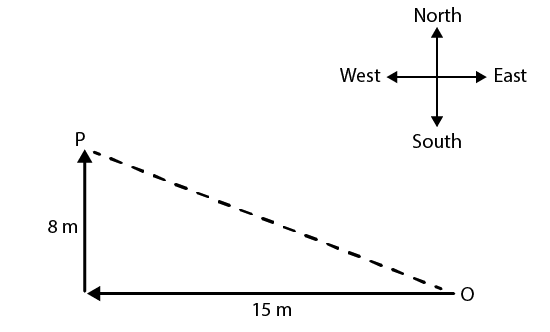Given a man goes 15 m due west and then 8 m due north
Let O be the starting point and P be the final point.
Then OP becomes the hypotenuse in the triangle.
So by using the Pythagoras theorem, we can find the distance OP.
OP2 = 15+ 82
OP2 = 225 + 64
OP2 = 289
OP = 17
Hence, the required distance is 17 m.
9. The foot of a ladder is 6 m away from a wall and its top reaches a window 8 m above the ground. If the ladder is shifted in such a way that its foot is 8 m away from the wall, to what height does its top reach?
Solution: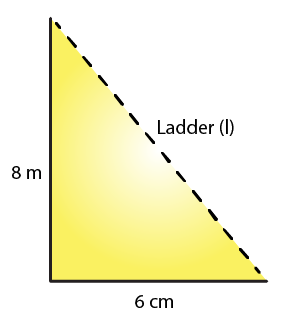Given Let the length of the ladder be L m.
By using the Pythagoras theorem, we can find the length of the ladder.
6+ 8= L2
L2 = 36 + 64 =100
L = 10
Thus, the length of the ladder is 10 m.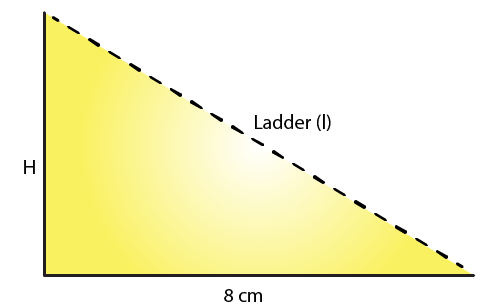Let the height of the ladder after it is shifted be H m.
By using the Pythagoras theorem, we can find the height of the ladder after it is shifted.
8+ H= 102
H= 100 – 64 = 36
H = 6
Thus, the height of the ladder is 6 m.
10. A ladder 50 dm long when set against the wall of a house just reaches a window at a height of 48 dm. How far is the lower end of the ladder from the base of the wall?
Solution: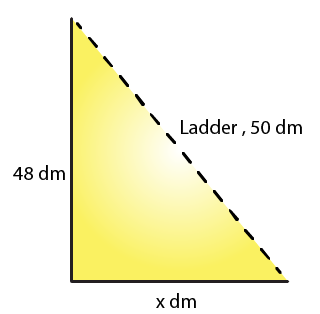Given that length of a ladder is 50dm
Let the distance of the lower end of the ladder from the wall be x m.
By using the Pythagoras theorem, we get
x2 + 48= 502
x2 = 50− 48
= 2500 – 2304
= 196
H = 14 dm
Hence, the distance of the lower end of the ladder from the wall is 14 dm.
11. The two legs of a right triangle are equal and the square of the hypotenuse is 50. Find the length of each leg.
Solution:
According to the Pythagoras theorem, we have
(Hypotenuse)2 = (Base)2 + (Height)2
Given that the two legs of a right triangle are equal and the square of the hypotenuse, which is 50
Let the length of each leg of the given triangle be x units.
Using the Pythagoras theorem, we get
x+ x= (Hypotenuse)2
x+ x= 50
2x= 50
x2 = 25
x = 5
Hence, the length of each leg is 5 units.
12. Verity that the following numbers represent Pythagorean triplet:
(i) 12, 35, 37
(ii) 7, 24, 25
(iii) 27, 36, 45
(iv) 15, 36, 39
Solution:
(i) The condition for Pythagorean triplet is the square of the largest side is equal to the sum of the squares of the other two sides.
372 =1369
122 + 352 = 144 + 1225 = 1369
122 + 352 = 372
Yes, they represent a Pythagorean triplet.
(ii) The condition for Pythagorean triplet is the square of the largest side is equal to the sum of the squares of the other two sides.
25= 625
72 + 24= 49 + 576 = 625
72 + 242 = 252
Yes, they represent a Pythagorean triplet.
(iii) The condition for Pythagorean triplet is the square of the largest side is equal to the sum of the squares of the other two sides.
45= 2025
272 + 362 = 729 + 1296 = 2025
272 + 362 = 452
Yes, they represent a Pythagorean triplet.
(iv) The condition for Pythagorean triplet is the square of the largest side is equal to the sum of the squares of the other two sides.
392 = 1521
15+ 362 = 225 + 1296 = 1521
152 + 362 = 392
Yes, they represent a Pythagorean triplet.
13. In △ABC, ∠ABC = 100°, ∠BAC = 35° and BD ⊥ AC meets side AC in D. If BD = 2 cm, find ∠C, and length DC.
Solution: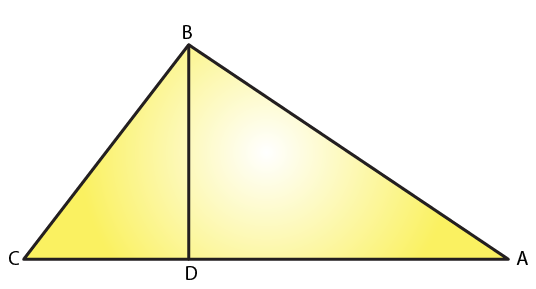We know that the sum of all angles of a triangle is 180o
Therefore, for the given △ABC, we can say that:
∠ABC + ∠BAC + ∠ACB = 180o
100o + 35o + ∠ACB = 180o
∠ACB = 180o –135o
∠ACB = 45o
∠C = 45o
On applying same steps for the △BCD, we get
∠BCD + ∠BDC + ∠CBD = 180o
45o + 90o + ∠CBD = 180o (∠ACB = ∠BCD and BD parallel to AC)
∠CBD = 180– 135o
∠CBD = 45o
We know that the sides opposite to equal angles have equal length.
Thus, BD = DC
DC = 2 cm
14. In a △ABC, AD is the altitude from A such that AD = 12 cm. BD = 9 cm and DC = 16 cm. Examine if △ABC is right angled at A.
Solution: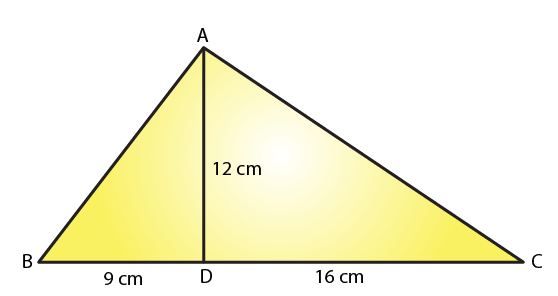Using the Pythagoras theorem, we get
12+ 16= AC2
AC2 = 144 + 256
= 400
AC = 20 cm
Using the Pythagoras theorem, we get
12+ 9= AB2
AB= 144 + 81 = 225
AB = 15 cm
Consider △ABC,
BC2 = 252 = 625
AB2 + AC2 = 152 + 20= 625
AB2 + AC2 = BC2
Because it satisfies the Pythagoras theorem, therefore △ABC is right angled at A.
15. Draw a triangle ABC, with AC = 4 cm, BC = 3 cm and ∠C = 105o. Measure AB. Is (AB)= (AC) + (BC)2? If not which one of the following is true:
(AB)2 > (AC)2 + (BC)2 or (AB)< (AC)+ (BC)2?
Solution: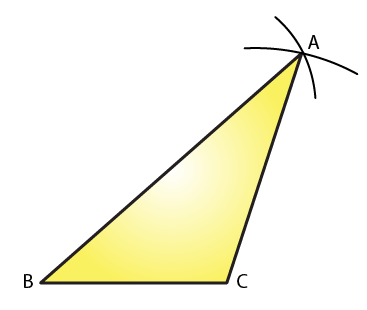Draw △ABC as shown in the figure with following steps.
Draw a line BC = 3 cm.
At point C, draw a line at 105o angle with BC.
Take an arc of 4 cm from point C, which will cut the line at point A.
Now, join AB, which will be approximately 5.5 cm.
AC+ BC2 = 42 + 3
= 9 + 16
= 25
AB2 = 5.52 = 30.25
AB2 is not equal to AC2+ BC2
Therefore we have
AB2 > AC+ BC2
16. Draw a triangle ABC, with AC = 4 cm, BC = 3 cm and ∠C = 80°. Measure AB. Is (AB)= (AC) + (BC)2? If not which one of the following is true:
(AB)> (AC)2 + (BC)2 or (AB)< (AC)+ (BC)2?
Solution: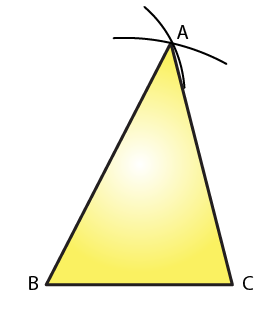Draw △ABC as shown in the figure with following steps.
Draw a line BC = 3 cm.
At point C, draw a line at 80° angle with BC.
Take an arc of 4 cm from point C, which will cut the line at point A.
Now, join AB, it will be approximately 4.5 cm.
AC2 + BC2 = 42 + 32
= 9 +16
= 25
AB2 = 4.52
= 20.25
AB2 not equal to AC+ BC2
Therefore here AB< AC+ BC2

Courtesy : CBSE